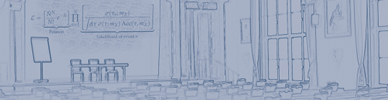#hadron2011

Jun 13 – 17, 2011
Künstlerhaus
Europe/Berlin timezone
Proceedings are now available online at <a href="http://www.slac.stanford.edu/econf/C110613/">eConf</a>

## New observations on hadron spectroscopy at BESIII

Jun 17, 2011, 10:40 AM
20m
Festsaal (Künstlerhaus)

### Festsaal

#### Künstlerhaus

talk Light Mesons

### Speaker

Ms Hongwei LIU (Institute of High Energy Physics)

### Description

With samples of 220 million $J/\psi$ events and 110 million $\psi^\prime$ events collected in the BESIII detector, $p\bar{p}$ mass threshold enhancement is studied. The enhancement is evident in $J/\psi$ radiative decay, which is consistent with BESII result. No significant narrow enhancement is observed in $\psi^\prime$ radiative decay. For $J/\psi\rightarrow\gamma\pi^+\pi^-\eta^\prime$ decay, the $X(1835)$, which was previously observed by BESII, is confirmed with a statistical significance that is larger than $20\sigma$ and the angular distribution of the radiative photon is consistent with expectations for a pseudoscalar. In addition, in the $\pi^+\pi^-\eta^\prime$ invariant mass spectrum, the $X(2120)$ and the $X(2370)$, are observed with statistical significances larger than ~$7.2\sigma$~ and ~$6.6\sigma$~, respectively. A new process $J/\psi\rightarrow\omega X(1870)\rightarrow\omega a_0\pi$ is also observed in $J/\psi\rightarrow\omega\pi^+\pi^-\eta$ decay.

### Primary authors

Ms Hongwei LIU (Institute of High Energy Physics) Prof. Shan JIN (Institute of High Energy Physics)

 Slides